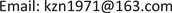﻿ 海洋生态损失价值的评估 Quantitative Analysis of Marine Ecological Loss

Vol.04 No.03(2017), Article ID:22491,6 pages
10.12677/AMS.2017.43014

Quantitative Analysis of Marine Ecological Loss

Xin Jia

Shandong Foreign Trade Vocational College, Qingdao ShandongReceived: Oct. 7th, 2017; accepted: Oct. 19th, 2017; published: Oct. 27th, 2017ABSTRACT

In the administration of marine resources, it is necessary to incorporate the value information of marine ecological loss caused by marine economic activities into decision-making. Therefore, it is necessary to evaluate the value of marine ecological loss. Based on the establishment of ten kinds of marine ecological losses, this paper uses different environmental impact assessment methods to establish the evaluation model of these marine ecological loss values respectively.

Keywords:Marine Ecological Loss, The Value of Marine Ecological Loss, Evaluation Model1. 引言

2. 海洋生态损失的形式

3. 海洋生态损失的评估

3.1. 海洋捕捞食品供给服务年损失价值

${V}_{fl}=\underset{i=1}{\overset{n}{\sum }}\left({q}_{1i}{p}_{1i}-{q}_{2i}{p}_{2i}\right)$  (1)

3.2. 海水养殖食品供给服务年损失价值

${{V}^{\prime }}_{fl}={r}^{\prime }RS$  (2)

3.3. 海洋原材料供给服务年损失价值

${V}_{ml}={r}_{m}{R}_{m}{S}_{m}$  (3)

3.4. 海洋气体调节服务年损失价值

${V}_{al}=\left(1.63{C}_{{\text{CO}}_{\text{2}}}+1.19{C}_{{\text{O}}_{\text{2}}}\right)XS$  (4)

3.5. 海洋环境容量服务年损失价值

${V}_{vl}=\underset{i=1}{\overset{n}{\sum }}{C}_{i}{X}_{i}S$  (5)

3.6. 海洋旅游娱乐服务年损失价值

${V}_{tl}={P}_{t}\Delta Q$  (6)

${P}_{t}=\frac{{V}_{T}}{{Q}_{T}}$ (7)

3.7. 海洋初级生产力服务年损失价值

${V}_{cl}={r}^{\prime }\frac{XE}{\delta }\sigma PS$  (8)

3.8. 海洋养分调节服务年损失价值

${V}_{yl}=\left({C}_{N}{Q}_{N}+{C}_{P}{Q}_{P}\right)S$  (9)

3.9. 海洋生境服务年损失价值

${V}_{el}={V}_{g}=\underset{i=1}{\overset{n}{\sum }}{C}_{i}$  (10)

3.10. 海洋生物多样性服务年损失价值

${V}_{dl}=PS$  (11)

Quantitative Analysis of Marine Ecological Loss[J]. 海洋科学前沿, 2017, 04(03): 96-101. http://dx.doi.org/10.12677/AMS.2017.43014

1. 1. 陈作志, 邱永松, 贾晓平, 钟智辉. 捕捞对北部湾海洋生态系统的影响[J]. 应用生态学报, 2008, 19(7): 1604- 1610.

2. 2. 纪大伟, 杨建强, 高振会, 贾永刚. 海洋溢油生态损害评估研究进展[J]. 水道港口, 2006, 27(2): 116-117.

3. 3. 王志霞, 刘敏燕. 溢油对海洋生态系统的损害研究进展[J]. 水道港口, 2008, 29(5): 367-371.

4. 4. 陈慧娟. 海水养殖对海洋生态环境的影响及其对策[J]. 福建水产, 2007(3): 47-50.

5. 5. 陈金平. 罗源牛坑湾围垦(填海)工程对海洋生态环境影响的分析[J]. 引进与咨询, 2006(10): 20-21.

6. 6. 张益民, 凌成健. 海洋工程对海洋生态影响及渔业资源损失的定量分析[J]. 海洋开发与管理, 2006(3): 110.

7. 7. 曾贤刚. 环境影响经济评价[M]. 北京: 化学工业出版社, 2003: 6, 138, 146.

8. 8. 李铁军. 海洋生态系统服务功能价值评估研究[D]: [硕士学位论文]. 青岛: 中国海洋大学环境科学, 2007.

9. 9. 曾贤刚. 环境影响经济评价[M]. 北京: 化学工业出版社, 2003: 6, 138, 147.

10. 10. 彭本荣, 洪华生, 著. 海岸带生态系统服务价值评估理论与应用研究[M]. 北京: 海洋出版社, 2007: 149.

11. 11. 张惠, 孙英兰. 青岛前湾填海造地海洋生态系统服务功能价值损失的估算[J]. 海洋湖沼通报, 2009(3): 34-38.

12. 12. 张帆. 环境与自然资源经济学[M]. 上海: 上海人民出版社, 1997.

13. 13. 吴姗姗, 刘容子, 齐连明, 梁湘波. 渤海海域生态系统服务功能价值评估[J]. 中国人口•资源与环境, 2008(2): 67.

14. 14. 田春暖. 海洋生态系统环境价值评估方法实证研究[D]: [硕士学位论文]. 青岛: 中国海洋大学环境工程, 2008: 45-46.

15. 15. 曾贤刚. 环境影响经济评价[M]. 北京: 化学工业出版社, 2003: 6, 127, 131-132.

16. 16. 张帆. 环境与自然资源经济学[M]. 上海: 上海人民出版社, 1997.